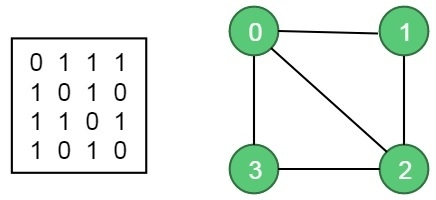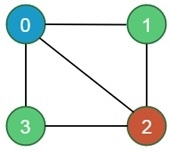# M-Coloring Problem

In this problem, an undirected graph is given. There is also provided m colors. The problem is to find if it is possible to assign nodes with m different colors, such that no two adjacent vertices of the graph are of the same colors. If the solution exists, then display which color is assigned on which vertex.

Starting from vertex 0, we will try to assign colors one by one to different nodes. But before assigning, we have to check whether the color is safe or not. A color is not safe whether adjacent vertices are containing the same color.

## Input and Output

Input:
The adjacency matrix of a graph G(V, E) and an integer m, which indicates the maximum number of colors that can be used.Let the maximum color m = 3.
Output:
This algorithm will return which node will be assigned with which color. If the solution is not possible, it will return false.
For this input the assigned colors are:
Node 0 -> color 1
Node 1 -> color 2
Node 2 -> color 3
Node 3 -> color 2## Algorithm

isValid(vertex, colorList, col)

Input − Vertex, colorList to check, and color, which is trying to assign.

Output − True if the color assigning is valid, otherwise false.

Begin
for all vertices v of the graph, do
if there is an edge between v and i, and col = colorList[i], then
return false
done
return true
End

graphColoring(colors, colorList, vertex)

Input − Most possible colors, the list for which vertices are colored with which color, and the starting vertex.

Output − True, when colors are assigned, otherwise false.

Begin
if all vertices are checked, then
return true
for all colors col from available colors, do
if isValid(vertex, color, col), then
add col to the colorList for vertex
if graphColoring(colors, colorList, vertex+1) = true, then
return true
remove color for vertex
done
return false
End

## EXample

#include<iostream>
#define V 4
using namespace std;

bool graph[V][V] = {
{0, 1, 1, 1},
{1, 0, 1, 0},
{1, 1, 0, 1},
{1, 0, 1, 0},
};

void showColors(int color[]) {
cout << "Assigned Colors are: " <<endl;
for (int i = 0; i < V; i++)
cout << color[i] << " ";
cout << endl;
}

bool isValid(int v,int color[], int c) {    //check whether putting a color valid for v
for (int i = 0; i < V; i++)
if (graph[v][i] && c == color[i])
return false;
return true;
}

bool graphColoring(int colors, int color[], int vertex) {
if (vertex == V)    //when all vertices are considered
return true;

for (int col = 1; col <= colors; col++) {
if (isValid(vertex,color, col)) {     //check whether color col is valid or not
color[vertex] = col;
if (graphColoring (colors, color, vertex+1) == true)    //go for additional vertices
return true;

color[vertex] = 0;
}
}
return false; //when no colors can be assigned
}

bool checkSolution(int m) {
int *color = new int[V];    //make color matrix for each vertex

for (int i = 0; i < V; i++)
color[i] = 0;      //initially set to 0

if (graphColoring(m, color, 0) == false) {    //for vertex 0 check graph coloring
cout << "Solution does not exist.";
return false;
}
showColors(color);
return true;
}

int main() {
int colors = 3;      // Number of colors
checkSolution (colors);
}

## Output

Assigned Colors are:
1 2 3 2